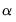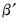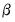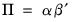User’s Guide : Multiple Equation Analysis : Vector Error Correction Models (VECMs) : VEC Data Members

VEC Data Members
Various results from the estimated VEC can be retrieved through the command line data members.
“Var Data Members” provides a complete list of data members that are available for a VAR object, but here, we focus on retrieving some of the VEC specific results.
For VEC models, the estimated coefficients are stored in three different two dimensional arrays: A, B, and C. A contains the adjustment parameters, B contains the cointegrating vectors, and C holds the short-run parameters (the coefficients on the lagged first difference terms).
The first index of A is the equation number in the VEC, while the second index is the number of the cointegrating equation. For example, A(2, 1) is the adjustment coefficient of the first cointegrating equation in the second equation of the VEC.
The first index of B is the number of the cointegrating equation, while the second index is for the variable in the cointegrating equation. For example, B(2, 1) is the coefficient of the first variable in the second cointegrating equation. Note that this indexing scheme corresponds to the transpose of.
The first index of C is the equation number of the VEC, while the second index is the variable number of the differenced regressor of the VEC. For example, C(2, 1) is the coefficient of the differenced regressor in the second equation of the VEC.
You can access each element of these coefficients by referring to the name of the VEC followed by a dot and coefficient element:
scalar a21 = var01.a(2,1)
scalar b21 = var01.b(2,1)
scalar c21 = var01.c(2,1)
To access the estimates of the full B matrix of coefficients, you may use the @cointvec data member:
matrix bmat = var01.@cointvec
The estimates of the adjustment coefficients A may be obtained using the @cointadj data member:
Thus, we can recover the estimate of thematrix using the command: